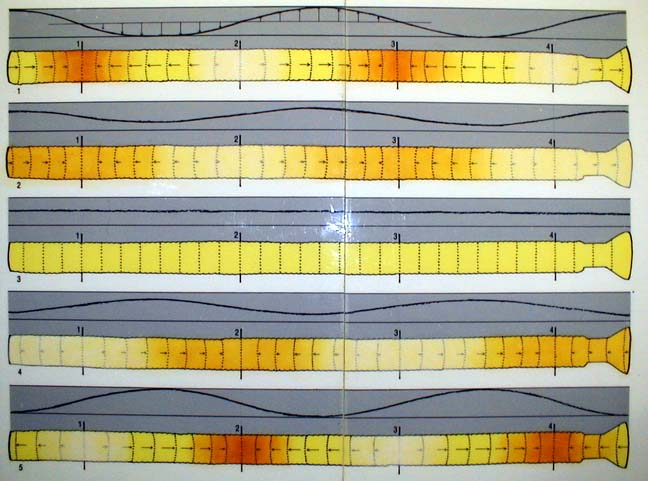Movie of airflow in a whirlyThe motion of air in the fourth harmonic resonance of a tube open at both ends. Each drawing is 1/8 of a cycle after the one above it.

Look at the illustrations above:

• The colors show the density of the air, orange is more dense, yellow atmospheric density, white less dense.
• The pressure is proportional to the density, so the color also shows pressure.
• The arrows and the dotted vertical lines show the displacement of the air.
• The sinusoidal black line in the gray field also shows the displacement of the air.

The cyclical motion of the air begins with the top image.

• Notice that the ends of the tube are at atmospheric pressure or density, yellow.
• The arrows indicating the displacement of the air from its equilibrium position are longest at the ends.
• At both ends the air is displaced to the right.
• Start at the left end and go right, you see a place where the density is greatest, and the displacement is zero. Here there are two vertical lines labeled 1. This is the first node of displacement.
• The black line above node 1 crosses through zero.
• To the right of node 1 the arrows point to the left and get longer away from the node.
• The arrows showing displacement are turned through 90 degrees and plotted on the line graph above.
The line shows the displacement of air in the tube.
• Notice the other nodes, they alternate from high density to low density.
• Arrows point toward high density nodes and away from low density ones.

In the second frame, an eighth of a cycle later, the dense regions of air are expanding into the less dense regions.

• The displacement in the second frame is less at every point than in the first.
• The colors are more muted showing less density contrast.
• The line graph has lower amplitude.
• The ends of the tube should still be yellow.

In the third frame, a quarter of a cycle after the first, every air parcel is back at its equilibrium position.

• The tube is at atmospheric density and pressure everywhere.
• The graph above is a straight line.

In the fourth frame the air has moved away from node 1 and toward node 2.

• Density differences are developing at the nodes.

In the fifth and final frame

• The arrows of displacement are longest at the ends but this time they point to the left, opposite frame 1.
• The nodes alternate high and low density but now node 1 which had been a high pressure node is now a low pressure node.
• The graph is a sinusoid as in frame 1 but it is out of phase, or upside down.

Summary

Note that the displacement at all of the nodes is always zero.

Note that the color change at the nodes is from dark orange to white, the greatest changes in density and pressure happen at the nodes of displacement.

Note that at the ends, the displacement arrows go from longest to the right, to zero, to longest to the left. Yet the color changes least. The ends are nodes of density or pressure change and antinodes of displacement change.

Sketch the sixth, seventh, and ninth frames yourself to test your understanding. (Answer below)

 Scientific Explorations with Paul Doherty © 2001 21 Feb 2001